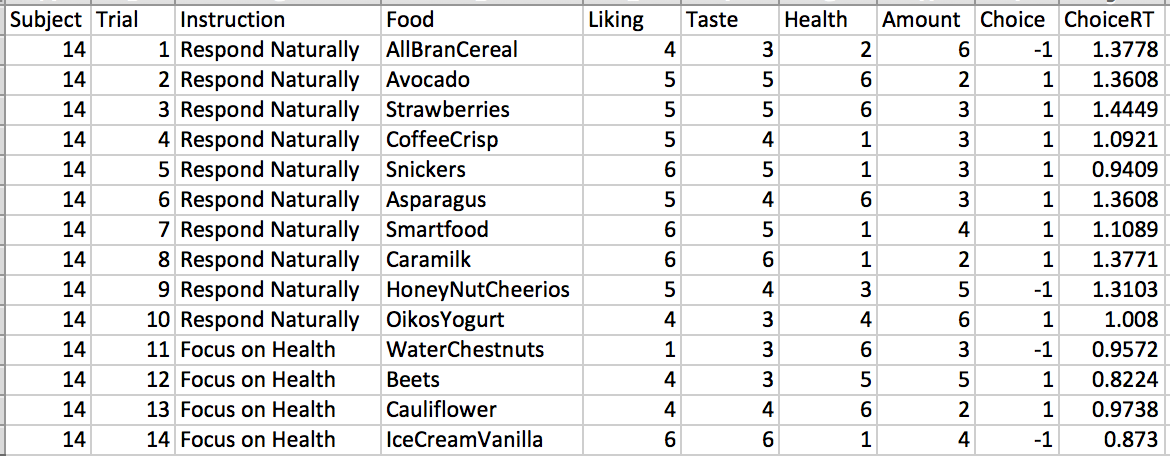Data Analysis Descriptive Statistics MATLAB makes calculating the mean, median, and mode of a set of numbers very simple. They also have a helpful tutorial on how to use these functions. I won't belabor the point here, but check out their demos. T-tests If you have the Statistics Toolbox (which typically costs extra in MATLAB) you can perform one-sample and paired t-tests using the function ttest, and two sample t-tests using the function ttest2. Correlations Use the corrcoef function to calculate a basic Pearson's r and its P-value. ANOVAs You can perform one-way ANOVAs, two-way ANOVAs, ANCOVA etc. using anova1, anova2, and the aoctool. I don't typically do these kinds of analysis in MATLAB, preferring R, but if you want to you can find more information about these tests here. Regressions There are a number of tools for estimating multiple regressions, including regress (which is simple linear regression), and glmfit (which allows you to calculate general linear models). Plotting Check out the bar function for creating bar graphs. To add error bars, use the errorbar function and use the '.' specifier for the line type. To create a scatterplot, use the scatter function. To add a regression line, check out the lsline function. Exporting data for use by other programs You may prefer to do your data analyses in another program besides MATLAB. With the workflow I've set up, and the way I log data during the experiment, I've found it helpful to write a script that allows me to flexibly process and write out the data (which is stored in a MATLAB-specific .mat format) for use by another statistics analysis package, such as R. In essence, I will create a universally-readable text file for each subject, where the columns represent data variables, and each row represents a single trial's worth of data for those variables. For the example study presented in these modules, that means that I might want a data table like this:This contains all of the relevant information about each trial: what food was shown, what instruction was the participant given (i.e. Respond Naturally or Focus on Eating Healthy), how much does the person generally like the food, how tasty and healthy was that food (as rated by the participant), what was the food's portion size, what choice did they make, and how long did they take to make the choice? I can then use this information to calculate conditional means, run regressions, or any other analysis of choice, by simply importing this text file into the stats program I prefer. The function I wrote to perform this task is called convertDataToText.m. return to main page